×

Search anything:

#### Deep LearningGet this book -> Problems on Array: For Interviews and Competitive Programming

## 2. The Challenge of Learning Rate in Optimization

In the process of training neural networks, the learning rate plays a vital role. It controls the step size at which the optimization algorithm moves along the loss landscape to find the minimum. A large learning rate can cause the optimization process to diverge, leading to overshooting the optimal point, while a small learning rate can cause slow convergence and might get stuck in local minima.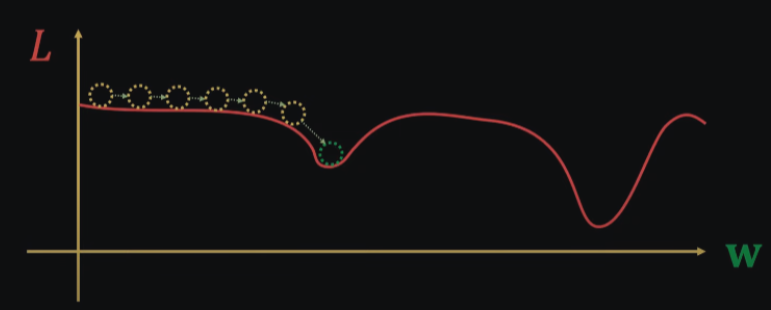Image illustrating the problem with too small learning rate, where the model progresses slowly and gets stuck in local minima.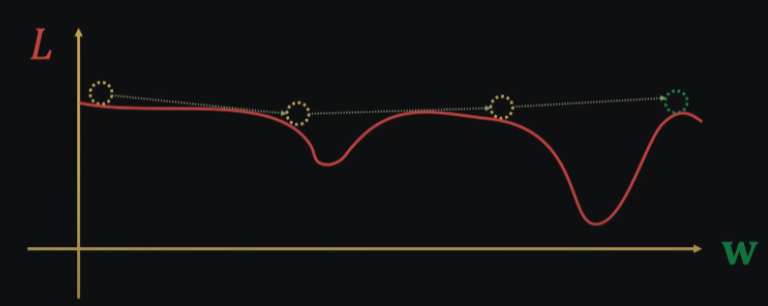Image illustrating the problem with too large learning rate, where the model doesn't converge.

Choosing an appropriate learning rate is often challenging since different layers of the neural network might require different step sizes due to the varying nature of gradients. Hand-tuning the learning rate can be cumbersome, especially when dealing with complex architectures.

In a nutshell SGD updates the model parameters according to the following formula: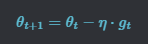Where the new parameters (theta_{t+1}) is equal to the old parameters (theta_t) minus the gradient (g_t) of the loss at those parameters times a learning rate (eta) which is constant. As mentioned previously this approach is particularly sensitive to changes in the learning rate.

AdaGrad aims at improving on SGD by enabling a change in the learning rate during the training. In Adagrad the learning rate is divided by the square root of the past gradients.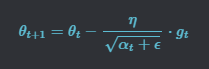The past gradients are calculated by initializing alpha = 0, then in every iteration the square of the gradient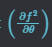is added to alpha so it will have the history of all past gradients.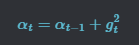Then the learning rate is divided by this term, changing the learning rate with each iteration (epsilon = 10^-8 is a term added to alpha to avoid zero division error).

There is however a problem with this approach as well, with every iteration alpha is increasing causing the learning rate to get smaller and smaller, causing training to stop.

In AdaDelta, instead of accumulating all the past squared gradients as in AdaGrad, it restricts the history of past gradients to a fixed-size window. This means it keeps track of a limited number of previous squared gradients rather than all of them. This limitation is achieved through an exponentially decaying average of squared gradients, denoted by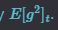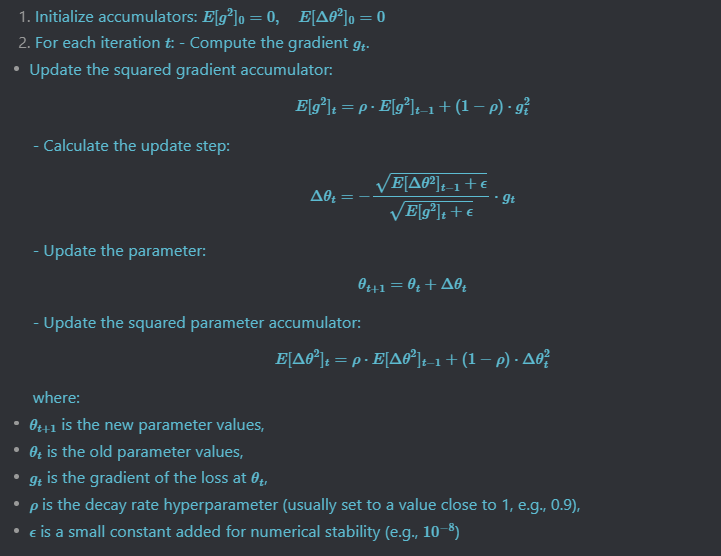• No Learning Rate Scheduling

AdaDelta doesn't require manual learning rate scheduling, as it adapts the learning rate dynamically based on historical gradients. This simplifies the hyperparameter tuning process and saves time during experimentation.

• Robust to Different Learning Rates

AdaDelta performs well with a wide range of learning rates, making it suitable for various neural network architectures without the need for extensive learning rate tuning.

• Mitigating the Vanishing/Exploding Gradient Problem

The adaptive learning rate helps to mitigate the vanishing and exploding gradient problems that often occur when training deep neural networks.

## 5. Conclusion

AdaDelta is a powerful adaptive learning rate optimization algorithm that addresses the challenge of tuning learning rates in traditional optimization techniques. By leveraging the historical information of gradients, AdaDelta adapts the learning rate for each parameter, making the optimization process more efficient and robust. Its ability to handle varying learning rates for different parameters and architectures makes it a valuable tool in the training of deep neural networks.

In summary of this article at OpenGenus, AdaDelta provides a significant step forward in the field of optimization algorithms, offering improved convergence and making it a popular choice among researchers and practitioners in the deep learning community. As the field of machine learning continues to advance, we can expect further developments and improvements in adaptive optimization algorithms like AdaDelta, contributing to the efficiency and effectiveness of training deep neural networks.